American Journal of Modern Physics
Volume 4, Issue 3, May 2015, Pages: 97-108

A Theoretical Confirmation of the Gravitation New Origin Having a Dipolar Electrical Nature with Coulomb Law Corrected

Ioan Has1, Simona Miclaus2, Aurelian Has3

1Land Forces Academy Sibiu, Technical Disciplines Chair, Rm. Valcea, Romania

2Land Forces Academy Sibiu, Technical Disciplines Chair, Sibiu, Romania

3University "C. Brancoveanu", Faculty MMAE, Economic Informatics Dep., Rm. Valcea, Romania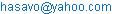(I. Has)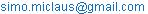(S. Miclaus)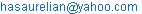(A. Has)

Ioan Has, Simona Miclaus, Aurelian Has. A Theoretical Confirmation of the Gravitation New Origin Having a Dipolar Electrical Nature with Coulomb Law Corrected. American Journal of Modern Physics. Vol. 4, No. 3, 2015, pp. 97-108. doi: 10.11648/j.ajmp.20150403.11

Abstract: The paper starts by analyzing the actual justification of the separation existing between electrical and gravitational forces, considering that for neutral bodies the electrical interaction force at long distances r, totally cancels as equal and opposed +/- forces FC, given by Coulomb law. Initially it was demonstrated that the type of the force FD, attraction or repulsion, between two electrical dipoles, having the same orientation, is given by the variation mode of the electrical forces FC with the r distance. In this paper one demonstrates that this electrical dipole force FD may exist at any distance r, by reciprocal orientation of any two dipoles. But such dipole force FD depends on a term in 1/r4 or greater power, being negligible compared to the gravitational force FN, which in Newton’s law depends on 1/r2. In order to obtain the principal term in 1/r2, for dipole interaction FD, it was necessary and sufficiently to admit a hypothesis which considers a new Coulomb law force FCC, as a series of terms of powers of r, including a new term of the form –ln r. With this corrected Coulomb law force FCC, for dipole interaction new force FDC, an expression having the principal term in 1/r2 results, as in Newton’s law. In order to verify the above hypothesis, numerical checking for the new dipole force FDC with first 4 terms of series was performed, utilizing actual electric permeability, the constant ε0 corrected, in all the terms. These calculations made for an astronomical distance (109m), showed a good agreement (relative ratio R=FN/FDC ≈ 0.626) between the force FN given by Newton’s law and the dipole force FDC obtained with the corrected Coulomb law. On the basis of this gravity theory, some important consequences result, such as the inexistence of the gravitational waves, of the black holes, of the space gravitational curvature, and of the big-bang. This gravity theory with more than 4 terms of series yield all of the four known forces in nature, so unifying them. The new dipolar gravity theory is physically possible only in quantum manifestation of the charges, and admitting the presence of a continuum media (a modern ether) as physical support of electromagnetic interactions.

Keywords: Coulombs Force, Electric Interactions, van der Waals Forces, Substance Polarization, Newtons Gravitation Force

1. Observations about Gravitational and Electrical Interactions

It is unlikely that the interaction forces acting between two particles or bodies at any distances r, and at any scales, in micro and macro universe, may be perfectly described by some mathematical simple laws. This affirmation is justified by the reality complexity or infinity and in the case of Coulomb’s law of electrical forces (1) and of Newton’s law of gravitational forces (2), affirmation is justified also by discrepancies between results of actual simple laws and some experimental observations reported in the past.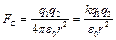; with k=1/4;             (1)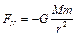(2)

Discrepancies concerning the Coulomb’s law is confirmed by modern experiments, which allow scientists to conclude that in the case of very short distances (such as between molecules, atoms, particles) the law is not precisely correct and it has not yet been proven in the case of long distances .

Concerning Newton’s law, there are some deviations which were found in the trajectories of cosmic bodies (comet movements, planet orbits, satellites trajectories, etc.) , while for very short distances (between atoms, particles) the law was not precisely checked. Many attempts to correct the deviations were made, starting with the utilization of exponential functions, of trigonometric functions, with terms containing the distance r at powers different from -2, or by admitting that the law (2) is valid in a non-Euclidean space. Three such attempts will be presented briefly.

Firstly, Boskovic (1749) proposed a more complicated correlation than the simple Newton’s law for the force between any particles. Boskovic’s curve  of force variation with the r distance begins with 2-3 oscillations which become a parabola at great distances. This means that the analytical expression of the force must contain a series of (1/r) powers.

Decombes (1913) admitted that gravitational force may be the result of an electro dynamical interaction between some pair of spectrons (groups of electrons), and then used the Ampere equation to calculate the force between two circular currents .

Another attempt was recently (1982) made by I. N. Popescu  which starts with the completing of the FN force from the Newton law, with a new term Fv representing a vortex force, established on the basis of fluid mechanics.

Based on the above observations, we can conclude that the two laws in the current forms (1) and (2) are not perfect and that they can be improved.

For a more accurate description of the infinite reality, including ether presence which will be considered throughout this paper, as we previously demonstrated, the most appropriate mode of forces description will be probable, a long or infinite series of successive powers of the r distance.

2. The Explanation for the Actual Absence of Electrical Interactions at Long Distances

Despite the fact that bodies are neutral, the +q and –q charges are in fact always separated in space at atomic scale and, consequently, every charge +q or –q will exert its electrical interaction force FC to infinite distance according to (1). The electrical force FC may be calculated directly by (1) or indirectly by mediation of the electrical potential φ and electrical field intensity E, both correlated by the equations :

E = grad j ; and F = q ×E                         (3)

Using the indirect method from (3), the electrical potential φ in a M point of space, for a continuous distribution ρ or for a discrete distribution of electric charges qi (Fig.1), will be: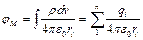(4)

Calculating the integral from (4), a series of powers of 1/r is obtained for φ , r being measured from the coordinates origin O: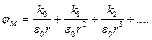(5)

In eq. (5), ki are various types of charge moments about O point and the behavior of φ at long distances will be given by the first non-null term. For a neutral body results k 0= Σqi=0 (the monopole momentum) and k1 ¹0. If all charges +q and –q can be symmetrically grouped around a certain point by twos as dipoles p with p = l (Fig.1), the second coefficient k1 (dipole momentum) will be: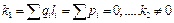(6)

When all dipoles can be grouped anti-symmetrically four by four, as quadrupoles, the result indicates k2=0 (quadrupole momentum), and k3 ¹0, and so on.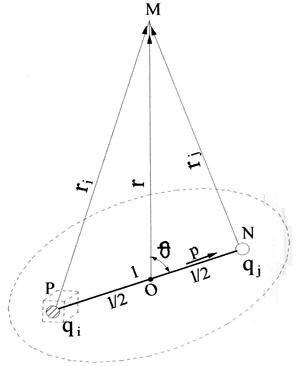Fig. 1. Elements for calculating the Coulomb force of two charges, in M point.

At present, it is accepted that macroscopic bodies have a random distribution of + and – charges, which will generate a random distribution of dipoles in any direction, and all coefficients ki from (5) are cancelled. This means that starting from small distances from any neutral body to infinite distances, the influence of the electric charges is considered today to be null.

Now, this electric potential fM (and FC) at long distances is considered to be null compared with gravitational potential.

3. Our New Hypothesis Concerning the Dipole Interactions at Long Distances

We will show that neglecting electrical potential even for distant bodies is not correct, because of reciprocal orientation of dipoles.

Consequently in this paper, analysis was considered of the interactions of electrical charges from the matter, which may appear at very long distances as terrestrial or astronomical ones, in fact in the range of common gravitational forces. These were logical and in accordance with Coulomb law (actual or corrected as in Chap. 7.) that in the presence of the electrical distinct +/- charges acting at infinite distance, anywhere in space will appear some interactions between distinct electrical +/- charges.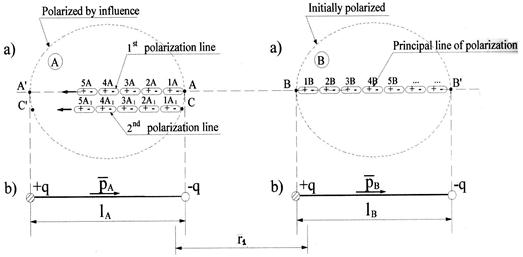Fig. 2. Interaction between two neutral bodies A and B constituted from atomic dipoles.

These electrical +/- charges will produce the polarization of neutral atoms, for all atoms comprising +/- charges, at any distance to the infinite (fig.2). Based on the reciprocal electrical interaction of any two dipoles, we can admit that all groups of the dipoles of two distant bodies will have, at a certain moment, the same orientation as it is obvious.

The dipoles must not be regarded exactly as classical permanent dipoles as presented in Fig.2, but as quantum atomic dipoles, capable of instantaneous interactions in any direction with another dipole. Admitting here as valid the superposition effects principle, an induced dipole p as in Fig. 2, is the result not of only one dipole influence, but many other quantum actions of other bodies being possible. In the next developments, the classic dipole will be considered for simplicity.

4. Actually Explanations and Admitted Expressions for the Forces at Short Distances in the Matter

The forces currently acting at short distances, namely at atomic, molecular, intermolecular, surface, or inter-particle distances, only forces of electrical nature are identified to be. Some of these forces are considered to be of long-range types, but they are localized at distances rarely exceeding 10-100nm . Even when the interaction of macroscopic particles, or when surface interaction was considered, this separation distance D was also about 100nm .

However, today these forces are considered to have a complex electrical nature, but they are not evaluated by the simple Coulomb law (1).

The various chemical bonds (ionic, metallic, and covalent) are considered to be intra-molecular attraction interactions, with the distance r~10nm. Today, they are thought to have an electrical nature, but they have more complex expressions than electrostatic forces (given by Coulomb’s law), as it was firstly proposed by Berzelius .

The van der Waals forces and hydrogen bonds are considered intermolecular electrical attractive forces appearing between neutral electrical systems with r~100nm. Debye and Keesom (1921) first showed, based on Coulomb law (1), that a polar molecule may induce a new dipole in a non-polar molecule having the polarizability a0. But starting from Coulomb’s law (1), a new term for the energy in r-6 (Debye induction) or in r-7 for the force, resulted .

Keesom obtained a new term of orientation for oscillating molecules, having the same form in r-6, but with different constants .

Later, London (1930) , based on quantum theory, considered the resonance between the electronic oscillators (with the frequency ν0 ), from two neutral molecules (atoms), having the deformability a. Between the two oscillators, the force is also given by Coulomb law (1). So London obtained a new dispersion potential φ for van der Waals forces, also explaining the light dispersion, depending on r-6. It is of the short-range type as was determined from real measurements for molecular van der Waals forces.

In order to account for the measured repulsion forces appearing at very short distances, the Lenard-Johnson potential was introduced containing beside the attraction term in r-6, a positive repulsive term in -r-12. The nature of these repulsive forces is not yet explained, but Boskovic’s assumption was confirmed so, for small distances.

In 1963, McLachlan  elaborated a generalized theory of van der Waals forces potential, including the earlier induction (Debye), orientation (Keesom) and dispersion (London) terms. So, starting again from the classical terms of van der Waals potential in r-6 and based on Coulomb law (1), McLachlan obtained a series of terms, each depending on different frequencies νi on polarizability of the molecules, on the medium and also on r-6.

We now remark that all presented theories of chemical and van der Waals forces accept these forces as having an electrical nature because they are based on classic Coulomb’s law in the form from eq. (1), but finally obtaining for their potentials, some terms in r-6 and in - r-12whose deduction presents some lack of clarity.

Attempts were also made to study interactions between macroscopic bodies as spheres, surfaces, cylinders, etc. of finite dimensions R situated at D distances. In these cases the interactive potential expressions, depending on 1/D for spheres or on 1/D2 for planar surfaces was obtained, but with D << R and starting again the deductions with the term in 1/D6 of van der Waals interaction potential. For "long" distances with D > R, the energy expression was obtained depending again on 1/D6, but the distances D were in fact short, on the order of 100nm , resting in the molecular domain and not at all in the astronomical domain.

We can see that in this short range distances, expressions of electric interactions depend on the r-6 and r-12 and not on the r-2 term as in Coulomb law.

But because no other force, (except FN force) was admitted to exist, we concluded that such terms are of electrical nature belonging to the Coulomb law which needs to be corrected consequently.

5. The Sense of the Interaction Force between Two Electric Dipoles

It is necessary in the next step of the theoretical analysis to establish the sense (orientation) of the interactive force appearing between two electric dipoles. It must determine if it is attraction or repulsion force, as function of the form of the electric force law.

For this, let us consider two distant bodies, A and B which are entirely polarized by influence in the same sense (Fig.2). The situation of two dipoles 1A and 1B originated in body A and B bodies respectively, identically oriented and equal to each other, with polar moment value q.l, is presented in Fig.3. The two possible cases of the F force variation with the distance r between two electric charges will be analyzed: a). the decreasing variation and b). the increasing variation.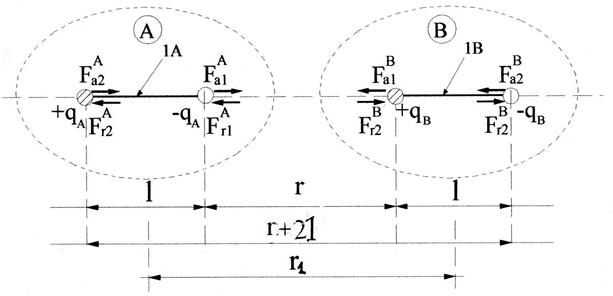Fig. 3. Forces acting between a pair of oriented dipoles 1A and 1B.

a). Initially, we suppose the interaction force Fa (attraction) and Fr (rejection) between electrical charges as being some vectors of central orientation decreasing with the distance r >> l, according to the laws of the general type, with n a natural number: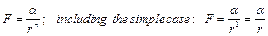(7)

From Fig.3, we now can calculate directly by simple algebra, for n=1, the total force upon each dipole, exerted by the other. Here we consider the + sign for force values having the sense of real force as in Fig.3. The attraction and repulsion force exerted by the charges +q, -q from 1B dipole upon 1A dipole utilizing the simple case (7') will be: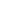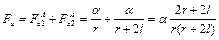(8)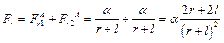(9)

We can rewrite the expression from the denominator from (8) as: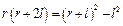(10)

From (10) admitting r >> l it results: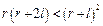(11)

Introducing (11) in (8) and comparing with (9) it results: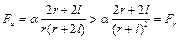(12)

From (12) it results that in the case of forces of the type as in (7'), decreasing (n= +1) with the r distance, the attraction force Fa will be greater than the repulsion force Fr and finally an attraction force between the two identically oriented dipoles results. This result is correct even if the power of r is any n >1, as it can easily be demonstrated.

b). Let’s suppose the force increases with the distance r, including a law of the form:

F = a' ln r                                    (13)

Again from Fig.3, the total forces upon 1A dipole utilizing (13) will be: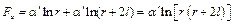(14)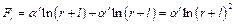(15)

Introducing (11) in (14) and comparing with (15), it results: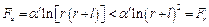(16)

From (16) it results that in the case of increasing forces of the type from (13), the repulsion force Fr between two identically oriented dipoles will be greater than the attractive force Fa and the resultant will be a repulsion force. This result is correct even if the increasing variation has the form F = a' rn with n>1, as it can easily be demonstrated.

The results from points a) and b) can be also obtained by a general approach utilizing the electric potentials j of the dipole.

6. The Force between two Far Dipoles, Admitting The Actual Coulomb’s Law

We chose the indirect calculation (as defined in Sec. 2) for the force FDx of an electric field E, upon a dipole, utilizing the electric potential j and using the relation :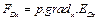(17)

In (17), EDx represents the electric field created by one of the two dipoles upon the other dipole in x direction (Fig.3). The field EDx created by 1A dipole, may be calculated as gradient of the dipole potential φDA. From the general equation for a neutral distribution (5), the dipole potential is given mainly by the second term and hence using (6) results: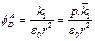(18)

where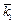is a coefficient of measure units. At the same time the equation from (17) may be rewritten using (3) for obtaining the force of 1A dipole, in x direction upon 1B dipole as: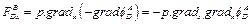(19)

Introducing (18) in (19) we get: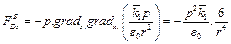(20)

From (20) it results that the force FDxB between two dipoles, regardless of the distance, depends in (20) on the distance r by a term in 1/r4 if Coulomb’s law is admitted valid in (5). The equation (20) differs essentially from (2) in 1/r2 and the dipole electric effects at large distances are negligible compared with gravitational force, today admitting to be null.

7. The Correction of Coulomb’s Law as FCC force by Adding Terms as New Powers of r

The dipole force FDxB from (20) varying with 1/r4 may be exerted at long distances r without limits, between any neutral electrically dipoles or bodies. It acts similar with the gravitational force between the same two bodies or dipoles, given by (2) but varying with 1/r2 .

At the same time, as it was mentioned in Sec. 1, the Coulomb equation (1) for electrical forces is not entirely valid for atomic or nuclear distances and was not verified experimentally for terrestrial and astronomical distances, where the weight of the term in 1/r2 may not give the total force.

Even observed movements of astronomic bodies exhibits deviations from the Newton equation (2), but the term in 1/r2 has the principal weight. Therefore, it seems logical to consider, as general law for these forces, not a simple power term but some series of powers terms in r around in 1/r2 term.

In order to bring the expression of FDxB from (20) closer to the form of Newton law from (2), we will introduce in eq. (20) some terms of such a series, around the term in 1/r2.

We observe that this fact should be possible if we intervene in the primitive equation of FDxB from (20), which is the equation (5). But we observe that φM from equation (5) is deduced utilizing the Coulomb’s law equation from (1), which need to be modified.

Therefore, we introduce a hypothesis admitting that it is necessary and justified from real behavior of electric charges to intervene upon the Coulomb’s law (1), which must be corrected in the next general form, by completing in both directions the missing terms of the series in 1/r powers for obtaining FCC as corrected Coulomb force: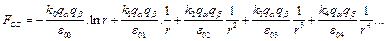(21)

But in the right part of (21) the series terms can continue, with terms in r-7 and r-13 as to obtain van der Waals forces and Lenard-Johnson potential.

The intensity of electric field E=FCC/qα of qa charge given by the newly corrected equation from (21) will be: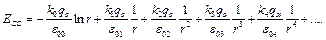(22)

In equations (21) and (22), the coefficients ki and ε0i represent new constants for measure units and respectively for medium or ether proprieties, similar with k and ε0 from (1), which should be determined in order to obtain the best correspondence between some experimental value for FCC or ECC , and the values calculated with (21) or (22). For k2 and ε02 , we can admit initially the actual values k=1/4p and respectively ε0. We can write the eq. (21) in a symbolic form: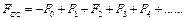(23)

In (23), the F2 term corresponds to classical force of Coulomb’s law FC from (1), but they will not be identical.

8. The Justification of the New Term in ln r

The first term -F0 in (23) depends on ln r and responds to the request of obtaining in (20) a term with r at the power –2. We note that this term -F0 in (23), depending on -ln r, indicates an increasing variation of –F0 with the r distance. In turn, all the other terms of the form A/rn, including F2, express a decreasing variation with r.

Here, the r from ln r is a simple number (dimensionless expression) of r, in selected units of length L in Coulomb law. So r has the sense as factor in L0 which precedes the term in L1, L2, L3 dimensions, in the term series proposed in (21) for corrected Coulomb law. The homogeneity of all the terms from corrected Coulomb law is given by measure units of ε0j constants, which differ.

According to the final observation from Sec. 5, a term of the form ln r gives an opposite sign (repulsion) to the electric force between two dipoles, compared to the sign of other terms of the form A/r n. As a consequence, the sign – for the term F0 was introduced in (21) and (23), in order to obtain an attractive force (as the gravitation) with (19) and then with (30), between two dipoles with the same orientation at large distances.

This forced algebraic operation of sign inversion in (21) or for the term F0 in (23) has, as a direct result, the inversion of the electric force sense given by this term F0 in (21), comparatively to the common Coulomb force Fc in (1) or to the other terms from (21) or (22). This means that, between two charges +q and -q, the –F0 term from (23) in ln r will give at long distances, according to (21), a repulsion force (inverse than the Coulomb force), while between two similar charges +q and +q (or -q and -q), the force will be an increasing attraction force with distance.

We note that also Eddington  obtained on the basis of his Fundamental theory, starting from hydrocules, a non–Coulombian interaction energy. This was given by an exponential term, which gives an attractive force between two protons situated at a distance of order of 5x10-13 cm.

Feynman  also sustains that the force between two protons is attractive for long distances, whereas for small distances it is repulsive but in accordance with Boskovic theory.

This first term in (21) or –F0 in (23) has the major weight in the case of great distances when the other terms become smaller, while - F0 rises slowly. This means that at long distances, the forces between the electrical charges will be given mainly by -F0 term, with the sign (-), opposite to the sign from the Coulomb law (1). But, at short distances (r<1,0), the same -F0 term will give a repulsion force between two charges of the same sign, while between two charges of opposite sign the force will be an increasing attraction force (like Coulomb force), by reducing the distance. But the weight of this term in (21) seems to be negligible at very short distances compared to the terms in 1/rn.

In (21) and in (23), other terms (F1, F3, F4...) were introduced, which have at this moment only the role of completing the expression of FC from (1) with the some missing powers of the infinite series. The necessity and the decision whether to maintain or not maintain these terms, or to include other terms in the final form of FCC will be taken with the aim of optimally matching eq. (21) with the experimental data at various distances ranges. The F7 term in r-7 may be identified with the van der Waals force and the term in r-13 with Lenard-Johnson potential, which both can miss for astronomical distances.

A first consequence of Coulomb’s law correction giving FCC will be analyzed in next Section but other possible consequences must appear as it is shown in Sec. 12.

9. Calculation of the Corrected Force FDC between Two Far Dipoles

The corrected electrical force FDC between two far dipoles, will be calculated by direct way defined in Sec.2 but utilizing the corrected Coulomb force FCC given by (21), respectively the corrected electric field ECC from (22), using the direct calculation as defined in Sec. 2, utilizing the equation (17).

For direct calculation it is necessary to establish the corrected electric field EDC of the dipole, from charge field ECC given by (22). This dipole electric field will be established by applying the principle of effects superposition, for the dipole charges +q and –q:

EDC=ECC+q -ECC-q                          (24)

We will note with rn and rp the distances (as scalars) from the two charges to the calculus point M (Fig.4), and we admit that for large r distances there will result small differences Δ and hence:

rp @ rn -Δ, and ln[(rn-Δ)/rn)] @(-Δ/rn)       (25)

Introducing (22) in (24) and renouncing at indices a and writing only the first 4 terms we get: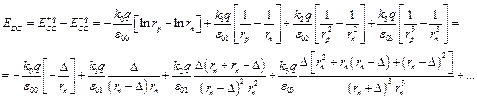(26)

In (26), neglecting the terms Δ, Δ2, Δ3 ... relatively to rn for large distances r comparatively with dipole length l, we obtain: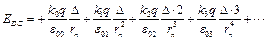(27)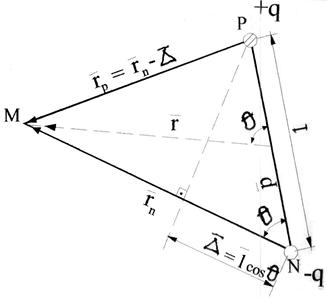Fig. 4. Calculation of the corrected Coulomb force, produced by a dipole p=ql.

We can also write from Fig.4 when θ≈0 for oriented dipole in M direction (as aligned dipoles):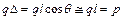(28)

Notating rn=r for large distances, and introducing (28) in (27) results: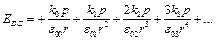(29)

Now we can calculate the attraction force FDC on the x direction between two identically oriented dipoles as in Fig.3, introducing (29) in (17) and obtaining: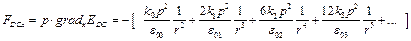(30)

In (30) we obtained the force FDCx on the x direction between two dipoles p and p, similarly and in parallel orientation, situated at a distance r, which always will be an attraction force because all the terms are negative. We observe the appearance in equation (30) of the first term in 1/r2 which is similar to Newton’s law from (2).

We now remark in (30) the new role of the first term in ln r from equation (21), which will give at long distances r, a dipole force F0 which will be greater than that given by the third term in 1/r2 (and also by the rest of the terms), which was solely considered as polarization factor in Sec.4. In this case the polarization effect of each neutral dipole given by ln r term will be stronger, and theoretically it rises increasing the r distance, in contrast with the Coulomb term in 1/r2 which decreases. We may consider that the forces exerted by each +q and –q charge, given by F0 from (23) or (21), will act effectively and strongly at long distances as polarization forces. These actions at atomic and subatomic scale probably develop discretely in time as quantum processes.

By the mechanism of reciprocal dipole orientation and polarization, and with such strong forces as F0, the matter gets a considerable power of reciprocal atomic and subatomic polarization even at long distances even astronomic, due to F0 term. Consequently, the atomic or even nuclear polarization will take place even at long distances between any two body, and the interaction force FDC from (30) will appear effectively and will act as the classical gravitational force FN from (2). We may expect that at long distances, as planetary, the first term from (30) will have the principal weight, but we must admit that the rest of the terms from the series, may also have a non-null influence.

At relatively short distances, atomic ones, the second or the rest of terms from right side of (30), must have an important weight. At very short distances, when Δ is not negligible compared with r, the relation (30) probably will be modified, and expressions such as van der Waals forces are justified.

10. Evaluation of Ether Constants ε0i for Corrected Coulombs Law by ρ Coefficients in FCC

In order to verify numerically the FDC force from (30) comparatively with FN force from (2), first we must evaluate the coefficients ki and especially ether constants ε0i from in FCC from (21).

In order to determine ad-hoc these coefficients, we will admit that the values of FC and FCC forces, given by (1) and (21), for the same real situation, must coincide: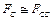For applying this condition, we will choose a possible real situation, with two charges qa and qb each composed of +20e and -20e (with e=1,6.10-19C) situated at a distance r=10-1m, corresponding to a domain in which the Coulomb’s law (1) was experimentally deduced by him. In this case the FC attractive force from (1) for ether (vacuum) medium will be: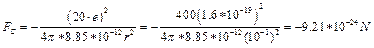(31)

In order to succeed in the easy application of eq. (21) to the same situation, we will limit this equation to a group of first 4 terms, the rest of terms we expect to be negligible at these relatively long distances, based on existing general observations at laboratory scale, but only some more precise dedicated measurements will validate it.

At this moment we have no information about the values of ether constants e00, e01 and e03. And even e02 from eq. (21) may have values different from actual electric permeability e0 from (1). The most reasonable and practical assumption is to admit initially as magnitude order, the same value for all ether permeability constants: e00=e01=e02=e03=e0=8,85.10-12 in measure units properly to every term.

Also we must consider k0= k1= k2= k3= k0= k=1/4p as in (1).

But in reality a difference of relative magnitude order, must exist between these 4 terms. So, the third term containing r-2 must have the quasi-total weight in FCC from (21), based on the results of Coulomb experiments carried out at the laboratory distance r≈10-1m, which validated at that time the law from (1).

In order to compensate in (21) the contribution of the 3 new terms, the value of the third term, should be diminished accordingly, compared to its value obtained in (31). We can do this redistribution of FC value, by means of 4 correction coefficients r0, r1, r2 and r3 that will be applied to the first 4 terms from (21), so we will differentiate the medium constants eoi which physically will give the weight of each term.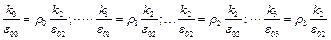(32)

Introducing (32) in (21) results: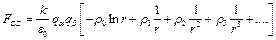(33)

These ρ coefficients must fulfill some conditions having at this moment only rational justifications as follows:

i). The first condition is that the main weight must be given to the 3-rd term from (21) as results of Coulomb measurements.

ii). The second condition must stipulate a gradual decrease of the ri coefficients at both sides of the principal term. This may be done admitting a "practical" experimental data error criterion, which assures the negligible relative weight of approx. 0,01 (1%) for the successive terms experimentally measured by visual observations. Otherwise, with relative differences greater than 0,01, even Coulomb and others should observe such deviations, from the proposed law as in eq. (1).

iii). The third condition must give finally the equalitythis assuring the final calibration of the coefficients from FCC by the real Coulomb force FC.

By some numerical trials we obtained the following correction coefficients fulfilling the above three conditions:

-for the 1st term: ρ0 =10 -4 ; for the 2nd term: r1 =10 – 2 ;

-for the 3rd term: ρ2 =0.9889; for the 4th term: ρ4 =10 – 3; : ρ5 =0,0                                          (34)

The correct ρi coefficients must be experimentally determined with accurate measurements of e0i at various increasing distances, even some astronomical.

Introducing in (33) values for q as in (31) and introducing (34) in (33) and reversing the signs in both members we get: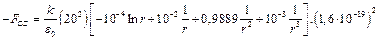(35)

Introducing values for ε0 and k in (35) we obtain: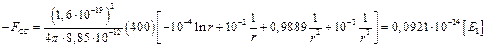(36)

Introducing in (36) the value r=10-1m as in (31) we obtain:-FCC =0.00002121*10-24 +0.00921*10-24+9.108+0,0921*10-24=9.209312*10-24N@9.21*10-24N=FC                   (37)

Therefore, the results for FCC from (37) and for FC from (31) are very close, FC ≈ FCC and we can utilize ρi coefficients from (34) in order to calculate dipole interaction FDCx in (30).

11. Numerical Verifications of Coincidence of the Force FDC between two Far Dipoles with the Gravitational Force FN

11.1. Dipole Evaluation and Calculation of Two Dipoles Force FDC

To calculate dipole interaction FDCx, we may consider any two bodies at long distance.

For simplicity, we will take as the two bodies, two distant atomic neutral dipoles of an arbitrary element from the middle of the Periodic Table. We will consider for simplicity, the dipole of a hypothetical atom having N=40, and Z=20 which means that it has 20 protons, 20 neutrons and 20 electrons.

Initially, we must evaluate the polarization distance b (l in fig.4) between the +q and –q charges in the considered polarized atoms, including neutrons. The order of magnitude of b is subatomic, and accordingly to Purcell  for short range polarization by medium electric field, b = 10-15 m, but for long range polarization we must take a shorter distance, with an order of magnitude, option reasonable, resulting:

l=b/10 =10-16 m                           (38)

We now can calculate the expression p2 appearing in (30) as: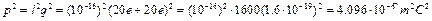(39)

Introducing (38) in (30) and noting with E the expression from parenthesis, it results: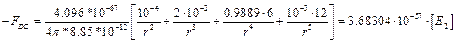(40)

11.2. Verification of the Force FDC between two Dipoles at Astronomical Distance

Now we choose for verification, a planetary distance for r when (2) is considered valid:

For r =1.000.000km=109 m, results from :                                               (41)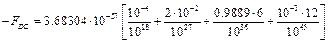(42)

-FDC = 3,68304•10-57[10-22+2•10-29+0,9889•6•10-36+12•10-48 ]= 3.68304*10-79N                                  (43)

Now we will calculate the gravitational force with Newton law from (2), (with G= 6,67.10-11m3kg-1s-2 and neglecting electron mass), for the masses of the same two dipoles of the same distance r from (41) results: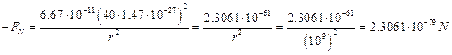(44)

From (43) and (44) results the relative ratio R:

R= FN / FDC =2.3061/ 3.68304 ≈ 0.62614           (45)

For a distance r =100.000km=108 m easy result from (40) a ratio R = 0.6258, very close to the precedent result from (45).

For greater distances r also in astronomical range the ratio R remains the same, about 0.63, as it is obvious from (40) and (44), but a value about 1.0 may be obtained by slightly modifying the l distance from (35) or modifying correction coefficients ρ from (32).

In  it was calculated for the same distance r a ratio R ≈2,50, neglecting de polarization of neutrons, but the case is not justified and consequently we considered in (39) the neutrons being polarized.

One remarks in (45) that the forces FN and FDC result of the same order of magnitude, in fact practically equal, for planetary or greater distances r in (41). We expect the results for R will fit about 1.0 more precisely, by utilizing experimental measurements for determining ε and k in (30) and even in (2) corrected.

11.3. Verification of the Force FDC between two Dipoles at Laboratory Distance

But in  for extra laboratory distance r =10m, from (40) and (44) the forces ratio R (amplified by 4 from neutron effect) it was obtained the small value: R=0,0161 (46)

And for laboratory distance r =10-1 m, from (40) and (44) we obtain: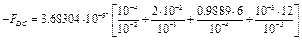(47)

-FDC =2.23*10 -52 N;                           (48)

And FN from results: -FN =2.3061*10 -59 N;      (49)

From (48) and (49) the two forces ratio R, become:

R=FN / FDC =2.3061•10-59/ 2.23•10-52 = 1.031•10-7   (50)

For short distances smaller than 10m, the results for FDC and FN in (50) differ more than in (45) and (46). For such short distances and even for molecular and atomic distances, other terms or new corrections for ether constants in (21) and in (30) or for l in (38), can intervene.

But for long distances, greater than 10m, the results for FDC and FN will give an R which will remain of the same order of values as in (45).

11.4. Determination of the Distance Where the Coulomb Corrected Force FCC Becomes Null

We can establish the distance r0 where the force FCC from (21) becomes null. For this we take from (21) or from (36) only the expression E1 and we find by trials, the distance r0. For a distance r0 = 60m we obtain E12: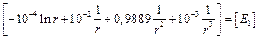(51)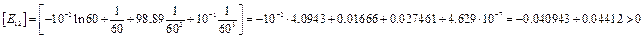(52)

And for a distance r0 = 70m we get E12: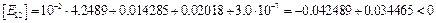(53)

This result indicates that with the precedent ether constants, at a distance r0 65m, takes place a sign change of corrected Coulomb force FCC for two +/-q charges, from attraction force to a repulsion force, beyond a distance of about 65m. At this r0 distance may produce also a change in behavior of gravitation forces but this value is only a preliminary value. This means that around r0 the force FCC becomes null and changes the sign, with his consequences.

Here we can note that some authors suggest that even the motion of swarms of animal or human crowds follow the Newton’s law, but our new law of gravity may be more appropriate in such a case [12, 13].

12. The New Theory of Gravitation Having Dipolar Electrical Nature and its Consequences

The work on this paper started from the justified supposition that a correlation must exist between electrical and gravitational forces, because each of these forces develops between the same matter particles and in the same transmission medium, the ether. Considering the previous positive results from Sec. 9, and based on precedent calculations from Sec. 11, a new theory of gravitational forces was initiated by authors, whose principal points and consequences are presented subsequently.

i). The first basic fact for the new Gravitational theory is the permanent separation in space, at atomic or nuclear scale, of the +q and –q charges, of all electrically neutral matter. As result even actual electrical Coulomb interactions forces FC, must exist at any distances, between separated charges +/-, which will create in any two bodies a general atomic polarization, with the same orientation.

But today it is not admitted to exist the dipole electrical forces at large distances, between two neutral bodies, probably by virtue of this body neutrality. However, But this concept is not entirely justified because such interaction forces will disappear only when all of the + and – charges will be completely superposed which is not the case in the real matter. This paper emphasizes that such polarization forces exist even with actual Coulomb law FC, but the resulted dipole forces depend on 1/r4, being negligible compared with gravitational force, depending on 1/r2.

ii). The second basic fact of the new theory consists of trajectories deviation from Newton law of cosmic bodies. There are also remarked discrepancies between Coulomb’s law forces FC and real forces at molecular scale, as van der Waals forces. This means that Newton’s and Coulomb’s laws are not perfect, and must be improved in order to furnish correct values for all the observed gravitational and electrical forces, at any distances r and at any time.

iii). The third basic argument for the new Gravitational theory is given by the successful correction of Coulomb’s law, by adding new terms as in Sec.7. Initially a general and logical correction of Coulomb’s law, FC, was made by completing the Coulomb’s equation in the form of FCC, as a series of terms in 1/rn, containing also a new term of the form –ln r. As a consequence of admitting and applying FCC, a new stronger dipole force FDC, having an expression also in 1/r2similar to gravitational force FN from Newton law was obtained. FDC is an attraction force between two neutral dipoles, depending also on r-2, as does the gravitational force FN. At this stage of the new theory development, the new force FDC may be considered physically different as nature and magnitude from Newton force FN, and acting simultaneously with this.

iv). The fourth basic argument for the new Gravitation theory is the numerical calculus results from Sec.8, for the new force FDC equivalent with FN.. In order to determine the magnitude of the new force FDC, in the paper it was made a numerical calculation of this attraction force FDC, for two atomic dipoles, situated at planetary distance. Because of lack of experimental measurements for data of Coulomb force FC at planetary distances, we utilized the electromagnetic constants ε0 and k from the actual Coulomb’s law FC, for estimating the corresponding constants of all the terms from Coulomb force FCC corrected law. These constants were calibrated for each term, with the available data for laboratory distances (FC values, ε0 and k constants actual values), by some rational correction coefficients ρ.

This numerical calculus revealed that this new dipole force FDC based on E-M constant ε0 has a value very close to the Newton gravitational force FN (ratio R≈1) based on gravitational constant.

v). The future confirmation of the above hypothesis, theory and results, will mean that any bodies, including cosmic ones, must manifest a global atomic and subatomic polarization of quanta nature, and consequently at this point, the gravitational forces do not exist as distinct natural forces, but may be regarded as dipole electromagnetic interactions, having an electric nature. As consequence, the mass has entirely an electromagnetic nature and not a gravitational one.

vi). Because the magnitude of the new force FDC is not negligible relative to the Newton force FN, even the two forces being practically equal, these two forces cannot exist simultaneously because superposition effect will give a exaggerated value, and consequently they must be the same force: the gravitation force FN consists in fact of electrical dipole interaction forces FDC. The Newton force FN must be replaced by electrical dipole interaction forces FDC and thus is accomplished a first real step of unification of two nature forces comprising gravitational and electrical forces.

vii). The above numerical result from Sec.11 which indicate that FDC= FN constitutes a first verification of the above proposed theory from this paper. This equality of the two forces can’t be a coincidence which is very improbable, but more probable it reflects a real fact. Other verifications, including experimental ones, or new analysis of some deviations from actual theories of gravitational FN and electrical FC laws, observed in many domains, including atomic and astronomic distances, may also confirm the above hypothesis and the resulted theory.

viii). If the polarization is absent at some elementary particles (electrons, protons, etc.), such isolated particle would not present above dipole interaction force, but they can interact with other dipoles by some modified forces, but starting also from FDC.

ix). Based on Coulomb’s corrected law giving FCC, improvements to the above results may be made in future upon determination of ε0 and k constants. And eventually by introducing new terms appropriate especially for short distances, for which in Sec. 10 an exact match between FN and FDC was not obtained. Development and possibly some improvements of these results, may be obtained in future by the quanta treatment of the electric dipoles, applied to the Coulomb corrected law FCC.

x). Now we must admit the general natural principle that a correct natural law, including FC, FCC, weak or strong nuclear forces, cannot be limited by any human hypothesis to be applied in all the space from zero to infinite distance, and at any time. In such conditions, the remaining two natural forces, the strong and the weak nuclear forces, may be deduced also from FCC (with new proper terms at right), therefore also these two forces will be reduced at electromagnetic forces, and so is accomplished a second real step of unification of nature forces comprising the above remaining tree forces. And so the grand unification is done.

xi). The possible criticism, namely that the corrected Coulomb force FCC gets very large values, even infinite values at r infinite, due to the term –ln r, which is, apparently, a paradoxical result, may be removed by admitting that +q and –q charges always are in pairs, from their apparition until their disappearance by reciprocal annihilation. Local imbalances of +q and –q charges may exist, and when FCC applies.

xii). Another possible criticism, may be the case of 2, 3 or more directions of interaction of a given dipole, when the FDC force should not act simultaneously in 2, 3 or more directions, in classical electromagnetism. This multi-directional interaction is still possible in quantum manifestation of the charges, and admitting the presence of a continuum media (as a modern ether) as absolute support of electromagnetic interactions.

xiii). Presence of this continuum media must yield as consequence, the inutility of special relativity theory principles, as was argued by our new interpretation of the Michelson experiment [14, 15, 16].

xiv). In addition to the above findings and results, the proposed correction of Coulomb’s law in the form of FCC, and ether presence must have other major consequences in physics, namely:

-- the modification of Gauss law of electric flux (derived from Coulomb’s law) and consequently one of the Maxwell’s equations of electromagnetic field will be modified, and as a result the actual solutions of electromagnetic field will change. The concept of the field loses its actual sense as EM forces support, being replaced by the ether.

-- the modification of the actual theory for the behavior of free ions (in cosmic space, in superior layers of the atmosphere, in particles accelerators, in plasma, etc.) when the gravitational forces do not appear anymore, and when corrected Coulomb’s law FCC acts, including this sign change ± at the particular distance of approx. 65m (Sec. 11.4).

-- the electromagnetic interactions based on the corrected Coulomb’s law (with more than 4 terms of series) are the only necessary and sufficiently to yield all of the four known forces in nature, so unifying them

-- the actual Newton’s law loses its object together with the attempts and experiments aiming to detect the gravitational waves.

-- in astrophysics, the so called big-bang moment, and the universal expansion of galaxies, will lose their theoretical basis, the actual gravitational forces FN.

-- in general relativity theory, the so called space/time entity and his gravitational curvature, will lose their starting basis, the actual gravitational forces FN.

-- in atomic or in nuclear physics and even in QM, the so called particles of all the kind including quarks, are in fact local stationary waves (vortexes) in the ether substance, each containing its specific energy which is manifested as particle mass, charge, spin, color, flavor, etc. by its proper complex vibration mode.

All these and other consequences of our Gravitational theory, concerning the electromagnetic interactions, as real support of so called gravitational forces, verified above by our numerical calculus, and of our correct interpretation of Michelson experiment , must be confirmed or refuted in the future by the physics community, by additional analysis and by interpreting or acquiring new experimental data.

Acknowledgements

The first author acknowledges the initial advice on the subject and encouragement of his late professor N. Barbulescu. He is sincerely grateful to prof. P. Mazilu from TUCB Bucharest for his rigorous lessons of rationality. He is also indebted to, Gen. prof. G. Barsan, to Col. prof. Al. Babos from LFA Sibiu, to prof. D. Stoicescu from ULBS Sibiu, to prof. D. Siposan from MTA Bucharest, to Ms. prof. Mirela Maier, to Ms. Laurel Sullivan from NHS, US, to Miss Veronica Has, and to Miss Kaylee Abend, US, for their continuous or final support. For the final developments of this theme the authors appreciate the support of the MEC (Grant under contract nr.05-D11-54/2005).

References

1. E. Luca, C. Ciubotariu, G. Maftei, G. Zet, A. Jeflea, and C. Pasnicu., Fizica, (in Romanian), Vol. 2, Editura Stiintifica, Bucharest 1996. p.65-70.
2. I. N. Popescu,, Gravitatia, (in Romanian), Editura Stiintifca & Enciclopedica, Bucharest 1982..p.25-78.
3. E. Vasiliu, Lumina- unda electromagnetica?, (in Romanian), Editura Albatros, Bucharest 1973. p.110-112
4. L. Decombes, Comptes Rendus, t.158, (1913), p. 940-943.
5. E.M. Purcell, Electricity and Magnetism, Berkeley, 1972. p. 59, 318, 328.
6. J. Israelachvili, Intermolecular and surface forces. Academic Press. London 1991. p. 7-260.
7. M. Born, Atomic Physics. (in Romanian), Editura Stiintifica, Bucharest 1973. p. 361.
8. McLachlan, Mol. Phys. 6, (1963), p. 423-427
9. A.S. Eddington, Fundamental Theory, Cambridge, 1949. p. 101-103
10. R.P. Feynman, Lectures in Physics, Electromagnetism and matter, Addison-Wesley, Mass.1965.p.159-160.
11. I. Has, S. Miclaus, A. Has. Physics Essays, Vol.21/4. (2008), p.303-312.
12. M. Romenskyy, V. Lobaskin. European Physical Journal B, Vol. 86, (2013), art. no. 91.
13. Y. Shang, R. Bouffanais. European Physical Journal B, Vol. 87, (2014), art. no. 294.
14. I. Has, Romanian Reports in Physics, Vol.52/10. (2000), p.775-789.
15. I. Has, S. Miclaus, A. Has.Physics Essays, Vol.23/2. (2010), p.248-257.
16. I. Has, S. Miclaus, A. Has. Optics, Vol. 3. Iss.4. (2014), p.24-32.

 Contents 1. 2. 3. 4. 5. 6. 7. 8. 9. 10. 11. 11.1. 11.2. 11.3. 11.4. 12.
Article ToolsAbstractPDF(590K)